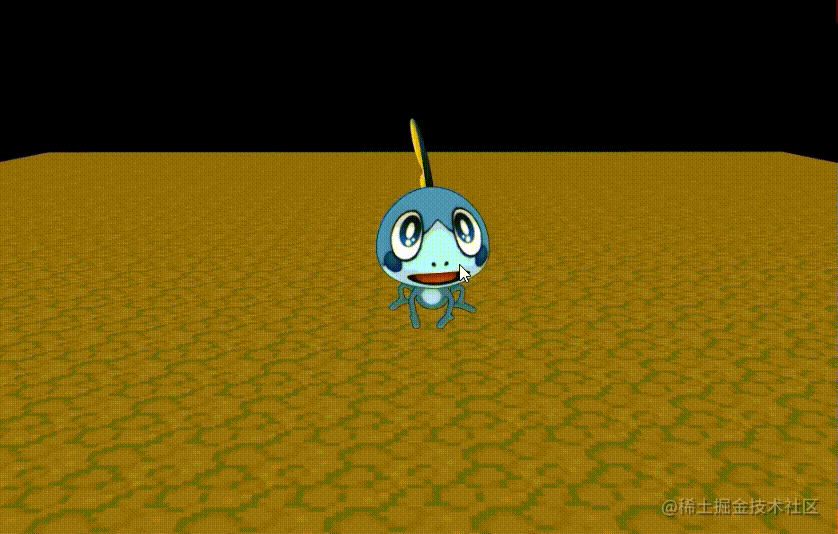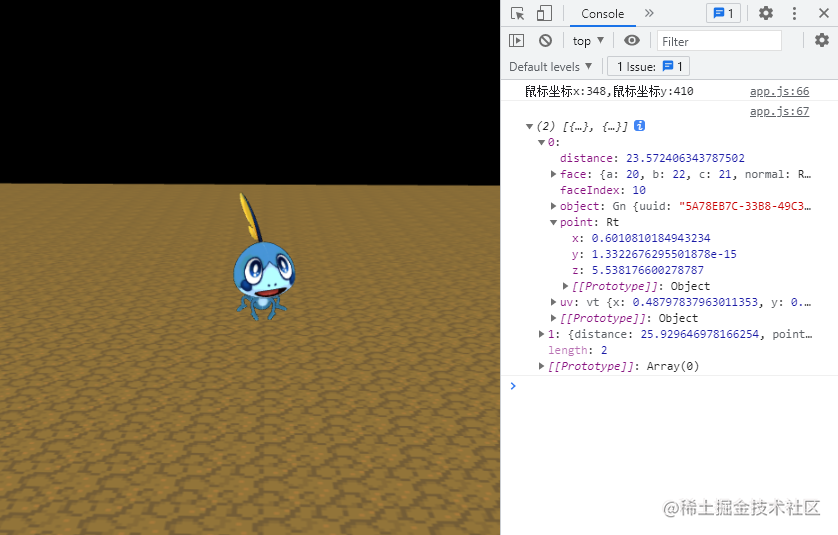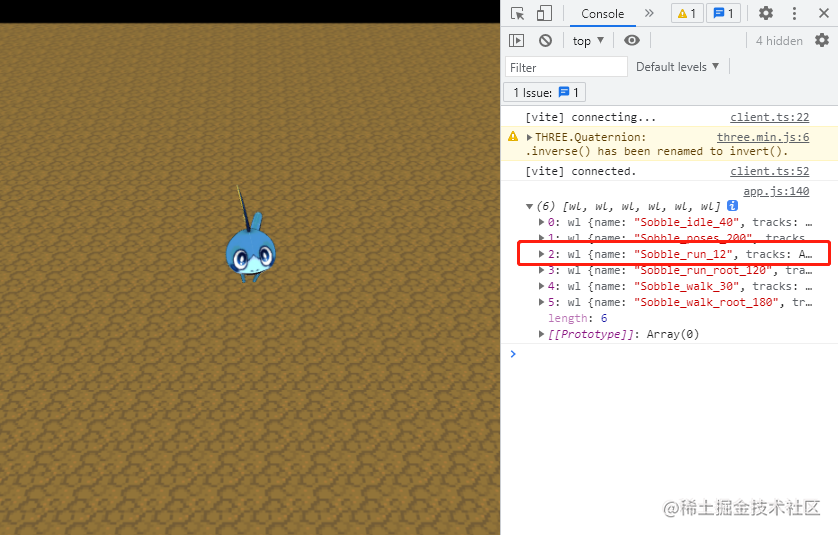## 前言## 正文

### 开发流程

2. 在主逻辑初始化，创建场景，灯光，摄像机，加载资源，绑定鼠标事件。
3. 资源导入后，创建地面和泪眼蜥，跳转大小和坐标及角色动画。
4. 鼠标点击实现泪眼蜥的移动和奔跑动画切换。

### 坐标的捕获与转化

``````bindEvent() {
// 其他要绑定的事件...
}

``````onMouseUp(e){
// 一些逻辑的判断，如果通过则让他执行捕获坐标让角色移动...
let vector = new THREE.Vector3();
vector.set(
(e.clientX / window.innerWidth) * 2 - 1,
-(e.clientY / window.innerHeight) * 2 + 1,
0.5);
vector.unproject(this.camera);
let raycaster = new THREE.Raycaster(this.camera.position, vector.sub(this.camera.position).normalize());
let intersects = raycaster.intersectObjects(this.scene.children);
if (intersects.length > 0) {
// 如果有坐标点，就存储下来
this.point = intersects.point;
// 宝可梦奔跑方法
this.pokemonRun();
}
}### 角色奔跑与移动

``````this.pokemon = gltf.scene;
this.animations = gltf.animations;
// ...
this.mixer = new THREE.AnimationMixer(this.pokemon);
this.mixer.clipAction(this.animations).play()
// ...

``````pokemonRun() {
if (this.timer) this.timer.pause();
this.mixer.stopAllAction();
this.mixer.clipAction(this.animations).play();
this.timer = new gsap.timeline({
defaults: {
duration: 0
},
});
let dx = this.pokemon.position.x - this.point.x;
let dz = this.pokemon.position.z - this.point.z;
let d = Math.sqrt(Math.pow(dx, 2) + Math.pow(dz, 2));
let angle = Math.atan2(dx, dz) + Math.PI;
this.pokemon.rotation.y = angle;
this.timer.to(
this.pokemon.position,
{
duration: d / this.speed,
x: this.point.x,
z: this.point.z,
onComplete: () => {
this.mixer.stopAllAction();
this.mixer.clipAction(this.animations).play();
},
}
);
}